# Fractions with Monomials Fractions with Polynomials Multiplying Dividing

• Slides: 31Fractions with Monomials Fractions with Polynomials Multiplying & Dividing Adding & Subtracting Equations 100 100 100 200 200 200 300 300 300 400 400 400 500 500 500 FINAL JEOPARDY! Click here once all questions have been answered!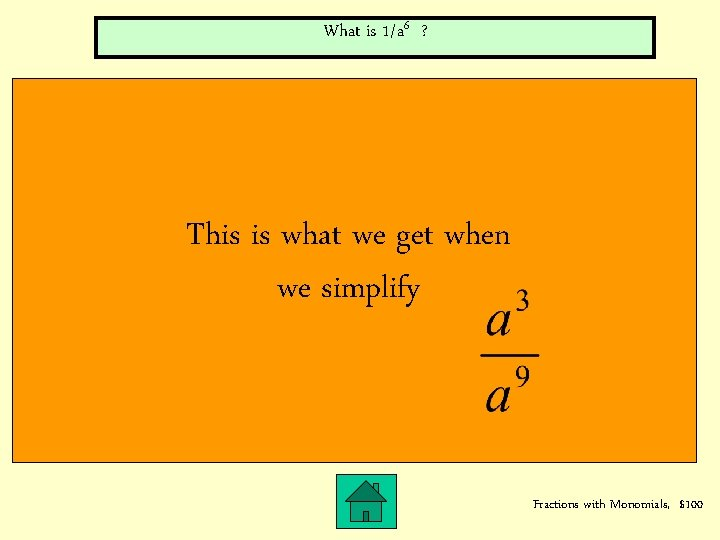What is 1/a 6 ? This is what we get when we simplify Fractions with Monomials, \$100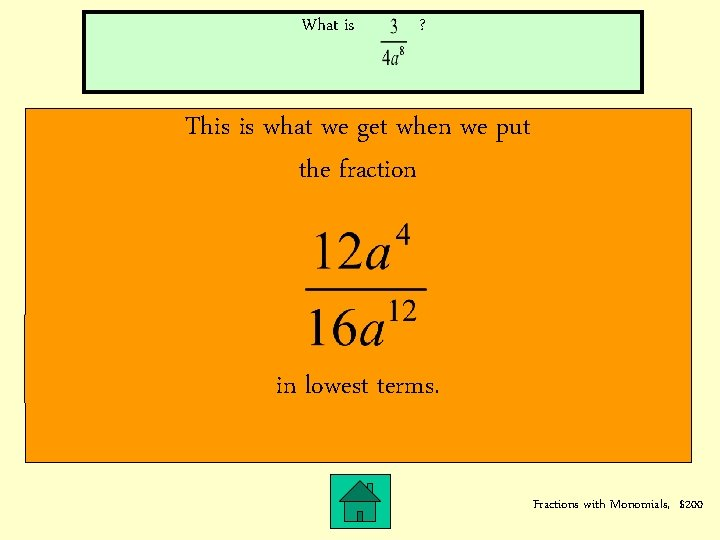What is ? This is what we get when we put the fraction in lowest terms. Fractions with Monomials, \$200What is ? This is what this fraction simplifies to: Fractions with Monomials, \$300What is ? SIMPLIFY Fractions with Monomials, \$400What is ? This is what this fraction simplifies to: Fractions with Monomials, \$500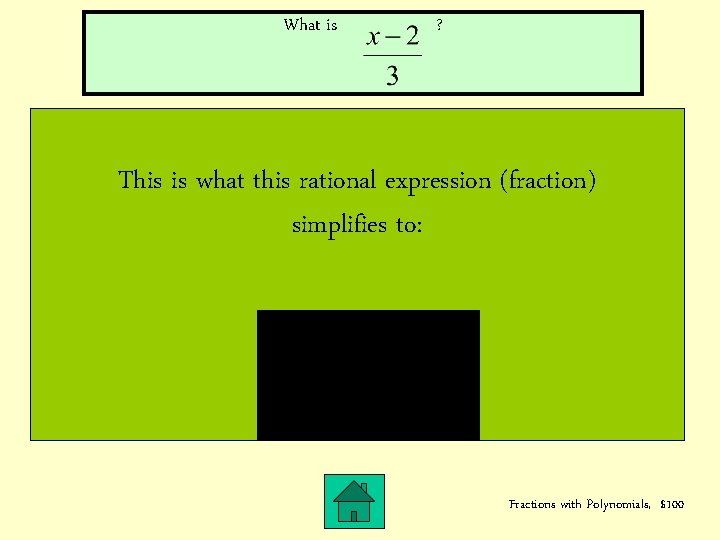What is ? This is what this rational expression (fraction) simplifies to: Fractions with Polynomials, \$100What is 9 ? This is the set of value(s) of x that make the fraction undefined: Fractions with Polynomials, \$200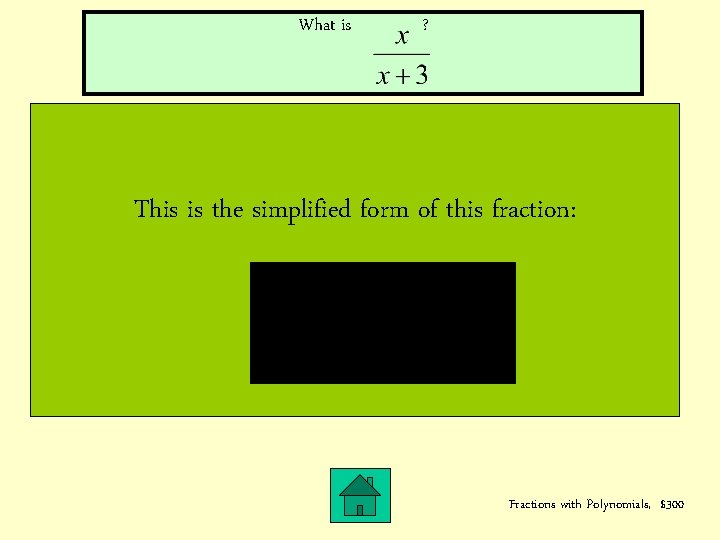What is ? This is the simplified form of this fraction: Fractions with Polynomials, \$300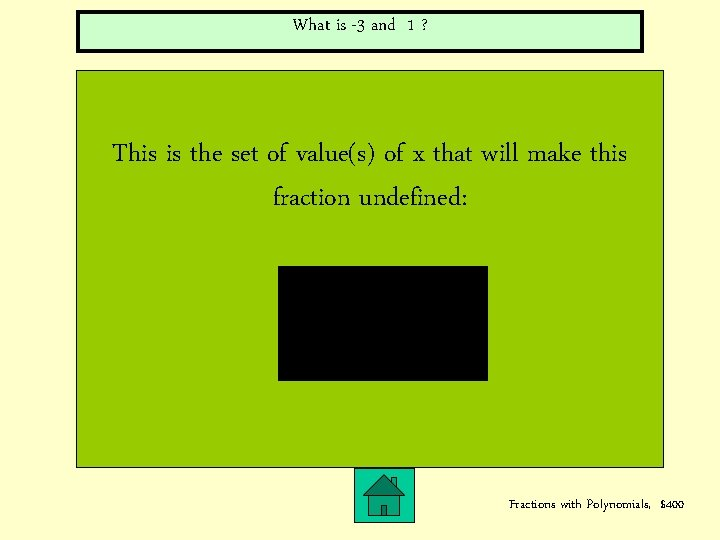What is -3 and 1 ? This is the set of value(s) of x that will make this fraction undefined: Fractions with Polynomials, \$400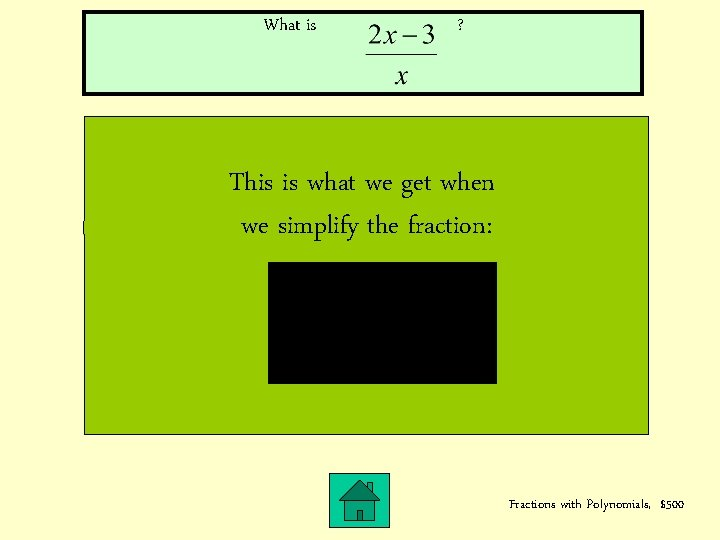What is ? This is what we get when we simplify the fraction: Fractions with Polynomials, \$500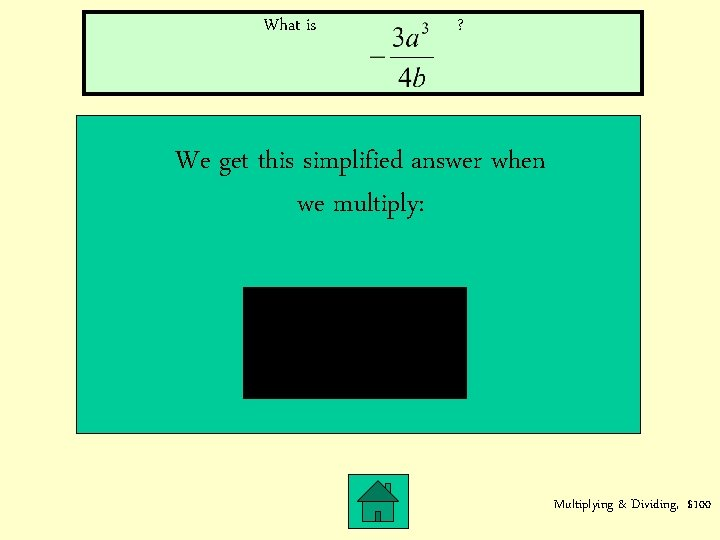What is ? We get this simplified answer when we multiply: Multiplying & Dividing, \$100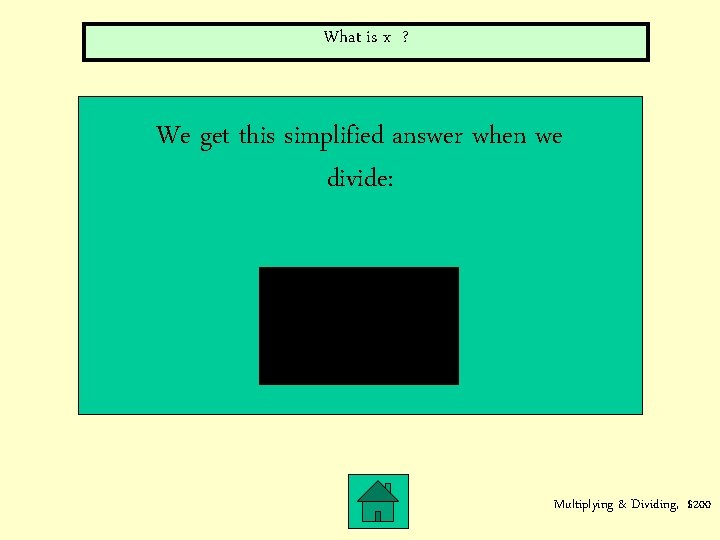What is x ? We get this simplified answer when we divide: Multiplying & Dividing, \$200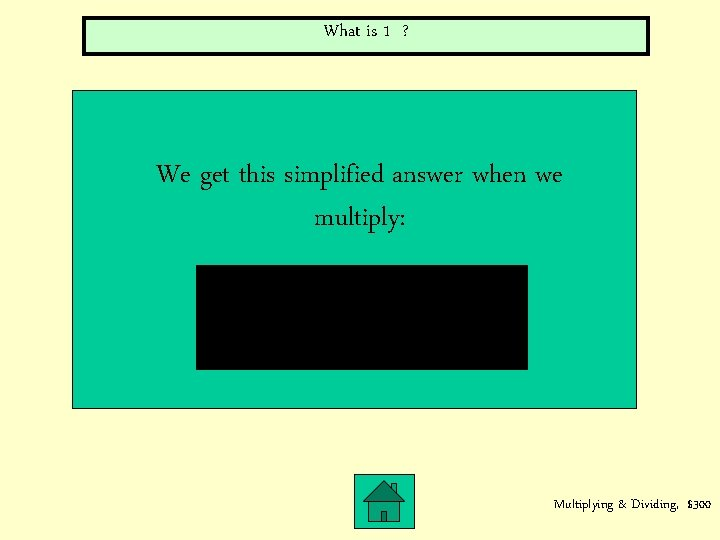What is 1 ? We get this simplified answer when we multiply: Multiplying & Dividing, \$300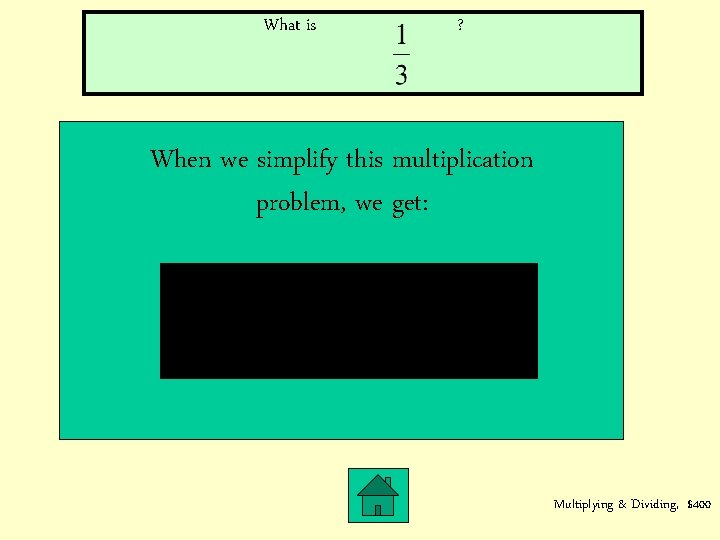What is ? When we simplify this multiplication problem, we get: Multiplying & Dividing, \$400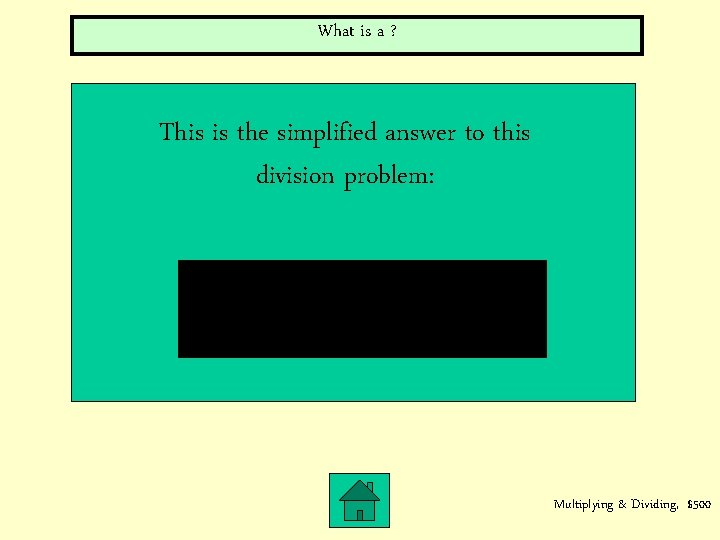What is a ? This is the simplified answer to this division problem: Multiplying & Dividing, \$500What is ? This is the answer to this addition problem, in lowest terms: Adding & Subtracting, \$100What is 1/p ? This is the simplified answer to the following problem: Adding & Subtracting, \$200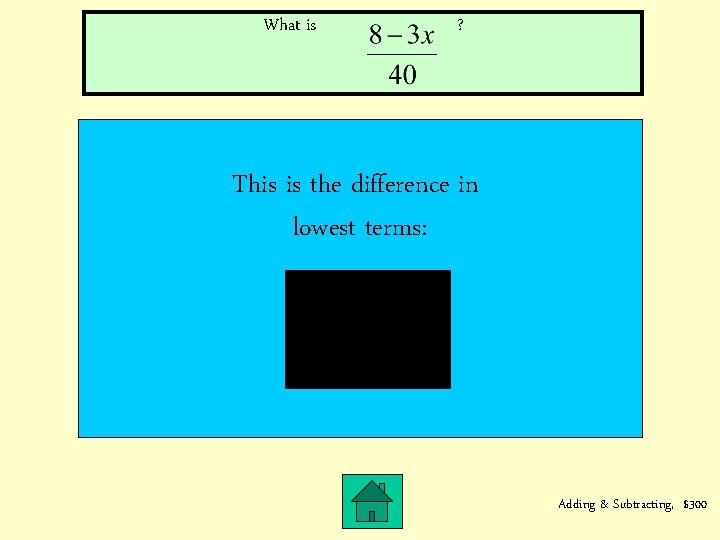What is ? This is the difference in lowest terms: Adding & Subtracting, \$300What is ? This is the simplified answer to the following problem: Adding & Subtracting, \$400What is ? This is the simplified answer to the following problem: Adding & Subtracting, \$500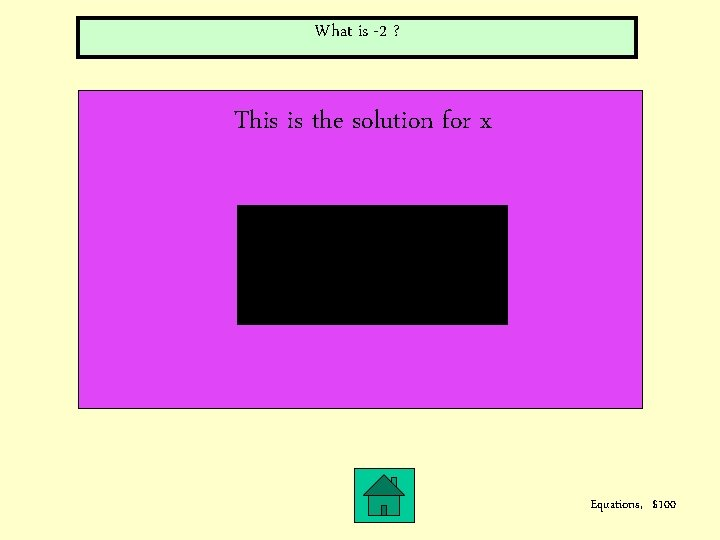What is -2 ? This is the solution for x Equations, \$100What is 2 ? This is the solution for x in the following equation: Equations, \$200What is 29 ? This is the solution for x in this equation: Equations, \$300What is 28/3 or 9. 333333 ? This is the solution for x in the following equation: Equations, \$400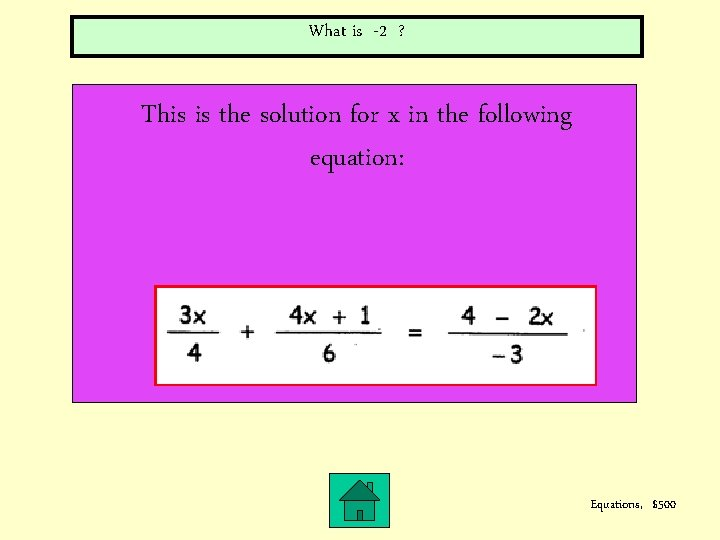What is -2 ? This is the solution for x in the following equation: Equations, \$500… s i y r o s. g r n e e t p o a i a t p c c r e a u r o y F Th f o g k n c i a y b f e li h t p n o m i w S o n r W r u o y e rit e g a w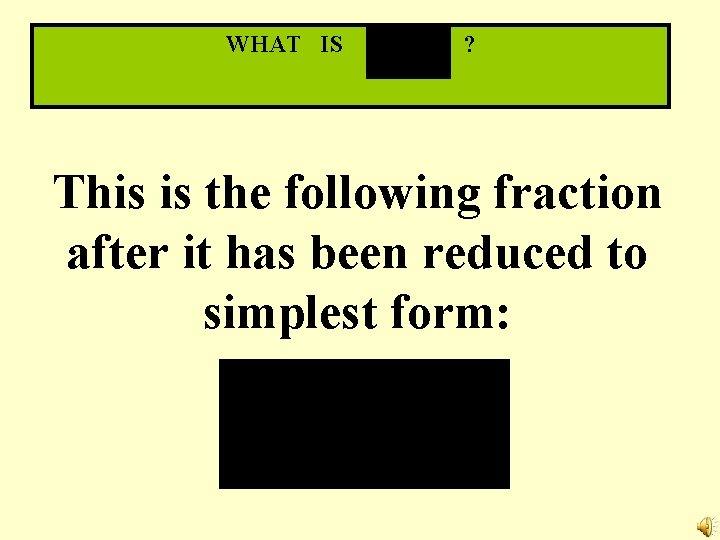WHAT IS ? This is the following fraction after it has been reduced to simplest form: